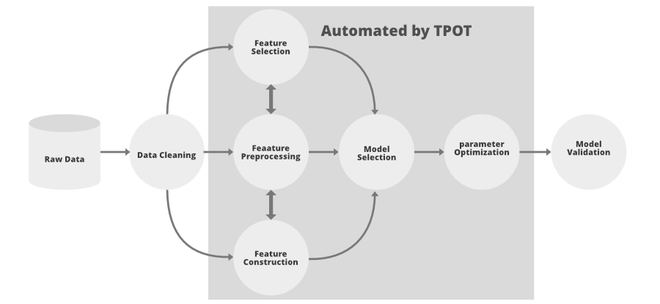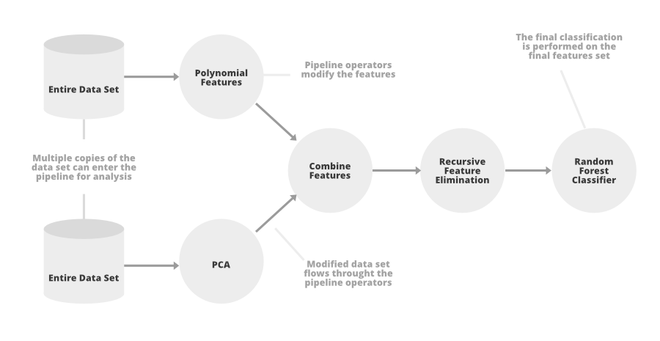Skip to content
Related Articles
Tpot AutoML
• Last Updated : 09 Mar, 2021

Tpot is an automated machine learning package in python that uses genetic programming concepts to optimize the machine learning pipeline. It automates the most tedious part of machine learning by intelligently exploring thousands of the possible to find the best possible parameter that suits your data. Tpot is Tpot is built upon the scikit-learn, so its code looks similar to the scikit-learn.Parts of ML pipeline automated by Tpot

Tpot uses genetic programming to generate the optimized search space, they are inspired by Darwin’s idea of natural selection, the genetic programming uses the following properties:

• Selection: In this stage, the fitness function is evaluated at each of the individuals and normalized their values, so that each of them has values between 0 and 1 and their sum is 1. After that, we decide a random number R b/w 0 and 1. Now, we keep those individuals whose value of fitness function is greater or equal to R.
• Crossover: Now, we can select the fittest individuals from above and perform crossover between them to generate a new population.
• Mutation: Mutate the individuals generated by crossover and perform some random modifications and repeat it for few steps or until we get the best population

Below are some important functions of Tpot:TpoT Pipeline

• TpotClassifier: module to perform automated learning for the supervised classification task. Below are some important arguments it takes:
• generations: number of iterations to run pipeline process (default: 100).
• population_size: number of individuals to retain genetic programming population every generation ( default 100).
• offspring_size: number of offspring to generate in each genetic programming iteration. (default 100).
• mutation_rate: mutation rate b/w [0,1] (default 0.9)
• crossover_rate: crossover rate b/w [0,1] (default 0.1) {mutation rate + crossover_rate <= 1}.
• scoring: metrics for evaluating the quality of the pipeline. Here scoring takes parameters such as Accuracy, F1 score etc
• cv: cross-validation method, if the given value is Integer, then it will be K in K-Fold cross-validation.
• n_job: number of processes that can be run in parallel (default 1).
• max_time_mins: maximum time Tpot allowed optimizing the pipeline (default: None).
• max_eval_time_mins: How many minutes TPOT has to evaluate a single pipeline(default: None).
• verbosity: How much information TPOT displays while it’s running. {0: nothing, 1: minimal information, 2: more information and progress bar, 3: everything} (default: 0)

• TpotRegressor: module to perform automated deep learning for regression tasks. Most of the arguments are common to above describe TpotClassifier. Here the only parameter which is different is scoring. In TpotRegression, we need to evaluate the regression, so we use parameters such as: ‘neg_median_absolute_error’, ‘neg_mean_absolute_error’, ‘neg_mean_squared_error’, ‘r2’

Both of the modules provide 4 functions to fit and evaluate the dataset. These are:

• fit(features, target): Run the TPOT optimization pipeline on the given data.
• predict(features): Use the optimized pipeline to predict the target values of an example/examples of features set.
• score(test_features, test_target): evaluate the model on test data and returns the most optimized score generated
• export(output_file_name): export the optimized pipeline as python code.

### Implementation

• In this implementation, we will be using Boston Housing Dataset and we will use ‘neg_mean_squared error’ as our scoring function.

## Python3

 `# install TPot and other dependencies``!pip install sklearn fsspec xgboost``%``pip install ``-``U distributed scikit``-``learn dask``-``ml dask``-``glm``%``pip install ``"tornado>=5"` `%``pip install ``"dask[complete]"``!pip install TPOT`` ` `# import required modules``from` `tpot ``import` `TPOTRegressor``from` `sklearn.datasets ``import` `load_boston``from` `sklearn.model_selection ``import` `train_test_split``import` `numpy as np`` ` `# load boston dataset``X, y ``=` `load_boston(return_X_y``=``True``)`` ` `# divide the data into train and test``X_train, X_test, y_train, y_test ``=` `train_test_split(X, y, test_size``=` `.``25``)`` ` `# define TpotRegressor ``reg ``=` `TPOTRegressor(verbosity``=``2``, population_size``=``50``, generations``=``10``, random_state``=``35``)`` ` `# fit the regressor on training data``reg.fit(X_train, y_train)`` ` `# print the results on test data``print``(reg.score(X_test, y_test))`` ` `#save the model in top_boston.py``reg.export(``'top_boston.py'``)`
```Generation 1 - Current best internal CV score: -13.196955982336481

Generation 2 - Current best internal CV score: -13.196955982336481

Generation 3 - Current best internal CV score: -13.196955982336481

Generation 4 - Current best internal CV score: -13.196015224855723

Generation 5 - Current best internal CV score: -13.143264025811806

Generation 6 - Current best internal CV score: -12.800705944988994

Generation 7 - Current best internal CV score: -12.717234303495596

Generation 8 - Current best internal CV score: -12.717234303495596

Generation 9 - Current best internal CV score: -11.707932909438588

Generation 10 - Current best internal CV score: -11.707932909438588

Best pipeline: ExtraTreesRegressor(input_matrix, bootstrap=False, max_features=0.7000000000000001, min_samples_leaf=1, min_samples_split=3, n_estimators=100)
-8.098697897637797```
• Now, we look into the file generated by the TpotRegressor i.e: the file contains code to read data using pandas and model for best regressor.
```#tpot_boston.py
import numpy as np
import pandas as pd
from sklearn.ensemble import ExtraTreesRegressor
from sklearn.model_selection import train_test_split

# NOTE: Make sure that the outcome column is labeled 'target' in the data file
tpot_data = pd.read_csv('PATH/TO/DATA/FILE', sep='COLUMN_SEPARATOR', dtype=np.float64)
features = tpot_data.drop('target', axis=1)
training_features, testing_features, training_target, testing_target = \
train_test_split(features, tpot_data['target'], random_state=35)

# Average CV score on the training set was: -11.707932909438588
exported_pipeline = ExtraTreesRegressor(bootstrap=False, max_features=0.7000000000000001,
min_samples_leaf=1, min_samples_split=3, n_estimators=100)

# Fix random state in exported estimator
if hasattr(exported_pipeline, 'random_state'):
setattr(exported_pipeline, 'random_state', 35)

exported_pipeline.fit(training_features, training_target)
results = exported_pipeline.predict(testing_features)```

### References:My Personal Notes arrow_drop_up